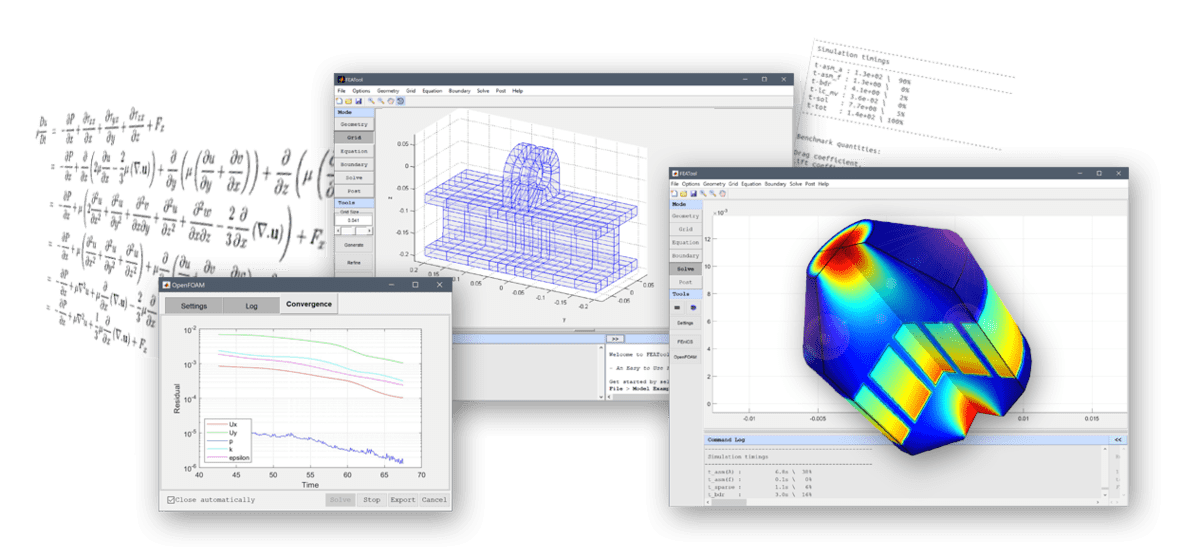# Modeling with Custom Equations in the FEATool GUIFEATool Multiphysics features an equation parser which allows users to very easily implement and simulate custom systems of equations. The goal as always with FEATool is to make it as easy as possible, the parser can therefore interpret equations written almost as one would on paper. For example a two dimensional Poisson equation

$\frac{\partial u}{\partial t} - D\cdot( \frac{\partial^2 u}{\partial x^2} + \frac{\partial^2 u}{\partial y^2} ) = 1$

can in FEATool simply be written as

u' - D*(ux_x + uy_y) = 1


The parser will process this equation string and decompose it into the constituents of the finite element weak formulation which is used by the assembly and solver routines. Moreover, other physics modes can similarly be changed to accommodate custom additions to the pre-defined equations.

An example showing how to implement a custom equation model, solving a Black-Scholes example equation for financial derivative modeling, can found in the Tutorial section of the FEATool User’s Guide

FEATool Custom Equation Tutorial